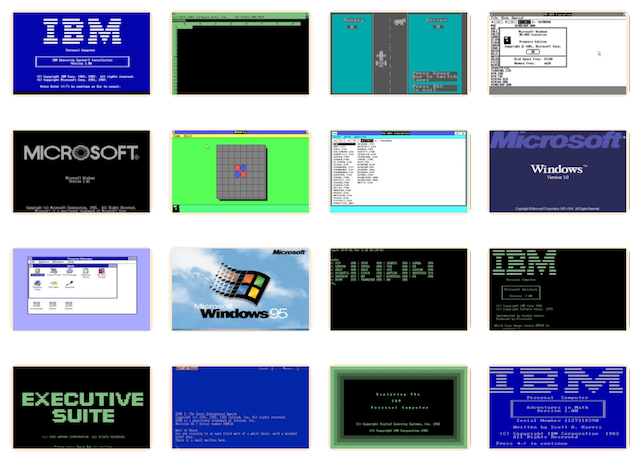# PCjs Machines

Home of the original IBM PC emulator for browsers.## SOFORECAST.BAS

By Jeff Parsons

This was a small program that my dad “commissioned” me to write in the late 70’s. He worked for many years at the Roseville Telephone Company in California, and the 1970’s were probably challenging and exciting times for the company, both in terms of keeping up with growth in the Roseville area and dealing with the rapidly changing technology, as the telephone industry transitioned from mechanical to digital switching.

I don’t recall the details, but this program appears to be a simple tool for forecasting some aspect of telephone customer activity (“SO” was an acronym, perhaps for “Service Orders”).

Challenger 1P
Speed:
Stopped

### Listing

``````5 FORC=1TO24:PRINT:NEXT
10 PRINT" ENTER NO. OF TYPES":PRINT:PRINT" OF DATA PER YEAR";:INPUTN
20 PRINT:PRINT" INPUT NAME FOR:":PRINT
25 FORC=1TON:PRINT" TYPE";C;:INPUTB\$(C):NEXTC
30 PRINT
99 X=1:DIMA(4,4,12),P(4,12),S(4)
100 PRINT" WHAT IS THE YEAR OF":PRINT:PRINT" DATA-BASE";X;
105 INPUTY(X):IFY(X)=0THEN150
110 FORT=1TON:PRINT
111 PRINT" ";B\$(T);"'S";:INPUTA\$:FORM=1TO11:FORC=1TO255
120 IFC=LEN(A\$)THENPRINT:PRINT" DATA ERROR":PRINT:GOTO111
125 IFMID\$(A\$,C,1)=";"ORMID\$(A\$,C,1)="/"THEN131
130 NEXTC
131 REM-SIFTING DATA-
135 A(X,T,M)=VAL(A\$):A\$=MID\$(A\$,C+1):NEXTM
140 A(X,T,M)=VAL(A\$)
145 NEXTT:X=X+1:PRINT:GOTO100
150 FORT=1TON:S(T)=0:FORM=1TO12:P(T,M)=0:FORX1=1TOX-1
155 P(T,M)=P(T,M)+A(X1,T,M):NEXTX1:P(T,M)=P(T,M)/(X-1)
156 S(T)=S(T)+P(T,M)
160 NEXTM,T:FORT=1TON:FORM=1TO12:P(T,M)=P(T,M)/S(T):NEXTM,T
170 FORC=1TO24:PRINT:NEXTC
180 INPUT" ENTER BASE YEAR";B
182 PRINT:PRINT" ENTER GROWTH RATE FOR:"
183 PRINT
184 FORY=1TON:PRINT" ";B\$(Y);"'S";:INPUTG(Y):NEXTY
190 FORT=1TON
215 Z=0:GOSUB500:IFZ=1THENT1=S:GOTO227
220 PRINT" WHAT WAS THE TOTAL":PRINT:PRINT" FOR BASE YEAR";B
225 PRINT:PRINT" (";B\$(T);"'S)";:INPUTT1
227 T(T)=T1:IFT>1THENFORV=1TO3000:NEXTV:GOTO230
228 INPUT" FORECAST YEAR";F
230 GOSUB235:NEXTT:GOTO264
235 TT=T1*(1+G(T)/100)^(F-B)
239 PRINT:PRINT:RESTORE
240 PRINT"  FORECAST YEAR";F:PRINT
241 PRINT"       (";B\$(T);"'S)":PRINT
243 FORM=1TO12STEP3:FORC=1TO3
250 FORC=1TO3:PRINTTAB(E(C));INT(TT*P(T,M+C-1));
260 NEXTC:PRINT:PRINT:NEXTM:RETURN
264 PRINT
267 PRINT" ENTER OTHER YEARS FOR":PRINT:PRINT" FORECASTING, BASED ON"
270 PRINT:PRINTB;"FIGURES...."
273 X1=1:PRINT
275 INPUT"   ";F(X1):IFF(X1)=0THEN280
277 X1=X1+1:GOTO275
280 IFX1=1THENPRINT:PRINT" TO BEGIN AGAIN,":PRINT:GOTO180
282 FORX2=1TOX1-1:F=F(X2)
283 FORT=1TON:T1=T(T):GOSUB235
285 FORV=1TO4000:NEXTV,T
286 NEXTX2
288 PRINT:GOTO267
300 END
400 DATAJAN,1,FEB,9,MAR,17,APR,1,MAY,9,JUN,17,JUL,1,AUG,9,SEP,17,OCT,1
410 DATANOV,9,DEC,17
500 FORX1=1TOX-1:IFY(X1)<>BTHENNEXTX1
505 IFY(X1)<>BTHENRETURN
510 S=0:FORM1=1TO12:S=S+A(X1,T,M1):NEXTM1
530 Z=1:PRINT:RETURN

``````